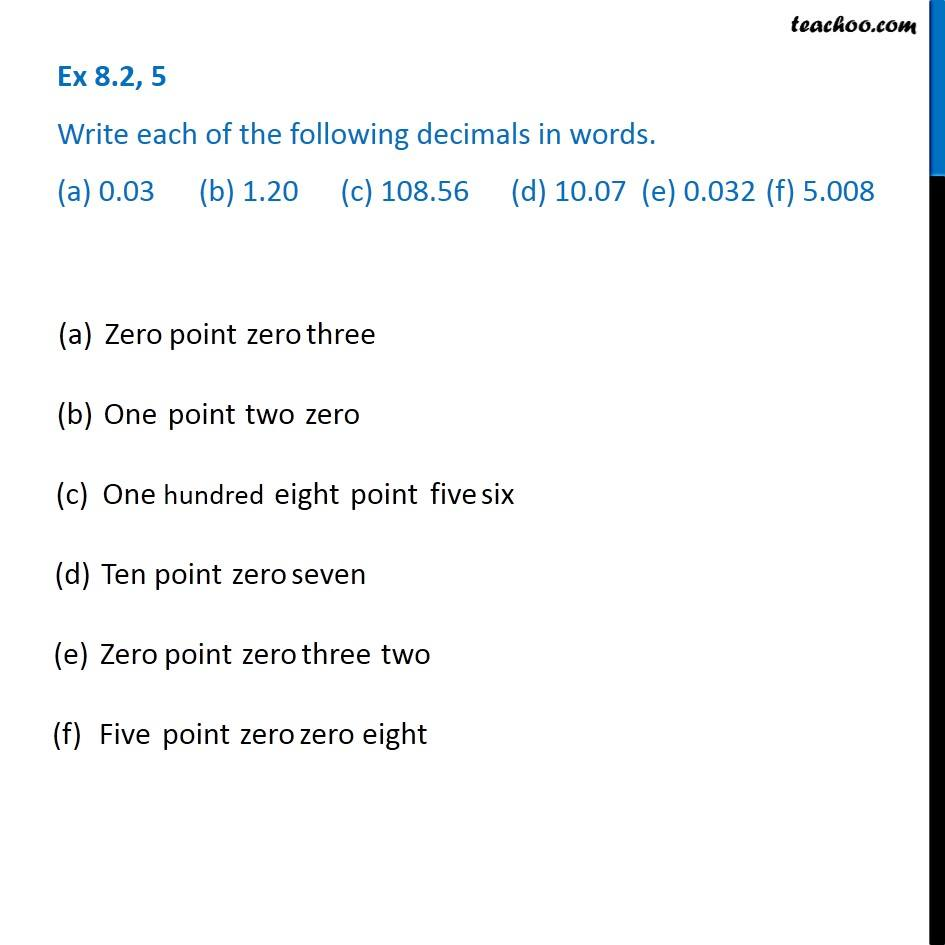1. Chapter 8 Class 6 Decimals
2. Concept wise
3. Words to decimals

Transcript

Ex 8.2, 5 - Chapter 8 Class 6 Write each of the following decimals in words. (a) 0.03 (b) 1.20 (c) 108.56 (d) 10.07 (e) 0.032 (f) 5.008 (a) Zero point zero three (b) One point two zero (c) One hundred eight point five six (d) Ten point zero seven (e) Zero point zero three two (f) Five point zero zero eight

Words to decimals